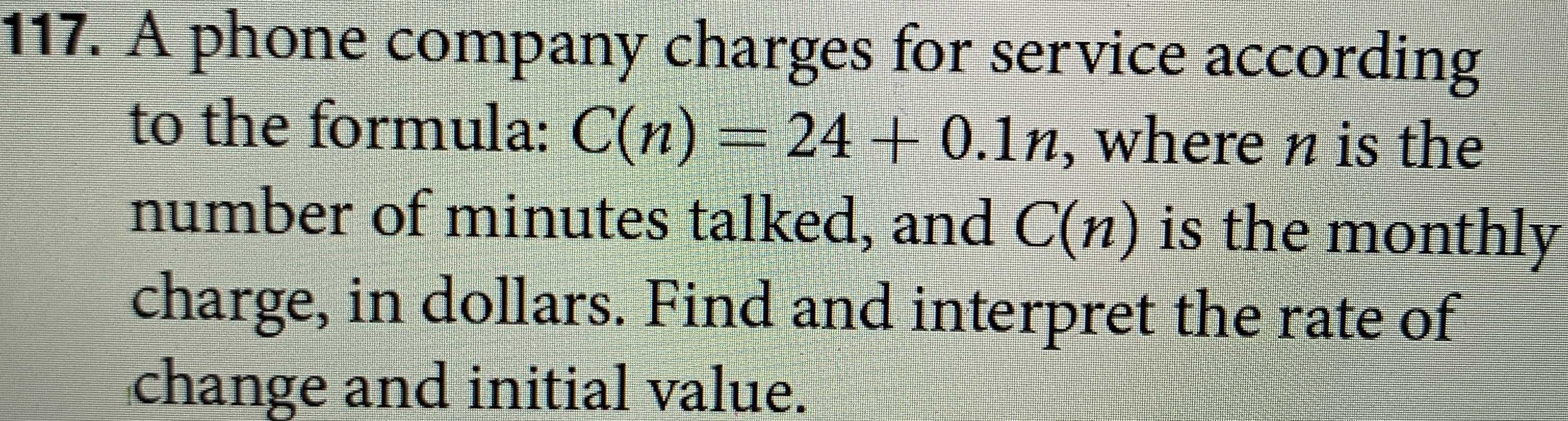### ¿Todavía tienes preguntas de matemáticas?

Pregunte a nuestros tutores expertos
Algebra
PreguntaA phone company charges for service according to the formula: $$C ( n ) = 24 + 0.1 n ,$$ where $$n$$ is the number of minutes talked, and $$C ( n )$$ is the monthly charge, in dollars. Find and interpret the rate of change and initial value.